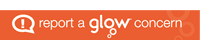# Physics

Physics is the study of the laws that govern how the Universe works, from relatively simple ideas like ‘the faster I go the more energy I have’ to the more advanced ideas – such as how space-time warps near massive objects. Physics has a strong link with Mathematics, and although the maths in Physics isn’t unduly difficult pupils will need the help of a calculator quite often as their course progresses.

Course outlines

S3

S3 Physics consists of 3 units : Dynamics Space and Electricity.

In the Dynamics unit we study motion and forces.  We build on knowledge gained in S2 to apply it to more challenging applications, including the famous S3 rocket activity.  We look at speed, acceleration, forces, friction and drag.

In the Space unit we take a more exploratory approach to our study.  We have a core unit which looks at key astronomical concepts such as planets, stars etc, but we also have significant student led aspects where we take the time to look at areas of interest to pupils.  The course is taught in multiple levels from s to 4 and even borrows material from Higher Physics towards the end of level 4.

In the electronics unit we look at the basic principles of electricity and then look to apply that through making circuits and conducting investigations.

S4

The National 4 and National 5 courses cover very similar material and both consist of three units : Dynamics and Space, Waves and Radiation and Electricity and Energy.  The main differentiator between the courses (other than assessment) is the level of mathematical demand.  National 5 has students working extensively with mathematical problem solving and applying maths learned in S3.  National 4 requires more elementary mathematical skills and is more accessible by those who would struggle in this area.  Both courses also have an assignment/AVU which forms of the course assessment.

Dynamics and Space covers speed, acceleration, forces, projectile motion, satellite motion and space exploration.

Electricity and Energy covers conservation of energy, kinetic energy, potential energy,charge, current, potential difference, electric fields, resistance, basic circuits and components.

Waves and Radiation covers wave motion, wave parameters(frequency, period, wavelength, speed), electromagnetic radiation, nuclear radiation fundamentals, applications and safety.

Higher

Higher consists of three units : Our Dynamic Universe, Electricity (half unit), Particles and Waves.  The course demands a higher level of problem solving and the concepts are correspondingly more advanced than National 5.   Students will work extensively on solving mathematical problems using more advanced techniques.  As with National 5, there is an assignment which forms part of the final course award.

Our Dynamic Universe covers forces, equations of motion, projectile motion, momentum, Newton’s law of gravitation, special relativity, origin and expansion of the universe, dark matter and dark energy.

Electricity covers electric fields, internal resistance, capacitors, semiconductors and junctions.

Waves and Radiation covers the standard model of particles, forces on charged particles, nuclear reactions and wave-particle duality.

Advanced Higher follows the structure of higher somewhat and has three units :  Rotational Motion and Astrophysics, Electromagnetism, Quanta and Waves.  There is also an assignment which forms part of the final grade.  Students are expected to use advanced mathematical skills developed in higher maths to derive and apply mathematical formulae in a more rigorous treatment of the subject.  The material studied, follows on logically from Higher.

Rotational Motion and Astrophysics covers derivation of kinematic relationships using calculus,  rotational motion, gravitation, general relativity, stellar physics,

Electromagnetism covers electrostatics, magnetic fields, capacitors, inductors and Maxwell’s equations.

Quanta and Waves covers quantum mechanics, particles from space, simple harmonic motion, travelling waves, polarization.

Subject online presence

We now have the department website which is in it’s infancy but is operational and accessible at www.forresphysics.co.uk.